# NCERT Solutions for Class 7 Mathematics Chapter 7 - Congruence of Triangles

##### Question 1:

When two triangles, say ABC and PQR are given, there are, in all, six possible matchings or correspondences. Two of them are (i) ABC $↔$PQR and (ii) ABC$↔$QRP.
Find the other four correspondences by using two cutouts of triangles. Will all these correspondences lead to congruence? Think about it.

Other four correspondences are:
ABC$↔$PRQ , ABC$↔$QPR
ABC$↔$RPQ , ABC$↔$RQP
All these correspondences will not lead to congruence.
If in any one of thse correspondences, the two triangles coincide then we say that the two triangles are congruent.

##### Question 2:

Two line segments are congruent if ___________ .

they have the same length.

##### Question 3:

Among two congruent angles, one has a measure of 70$°$; the measure of the other angle is ____________ .

70$°$

##### Question 4:

When we write $\angle$A = $\angle$B we actually mean ____________.

m$\angle$A = m$\angle$B.

##### Question 5:

Give any two real-life examples for congruent shapes.

(i) Take two stamps of same denomination.
Place one stamp over the other. We observe that one stamp covers the other completely and exactly. This means that the stamps are of the same shape and same size.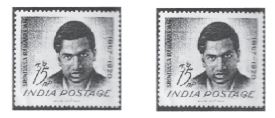So, two stamps used are congruent to one another.
(ii) Take two blades of the same brand. When we place one blade over the other; we observe that one blade covers the other blade exactly. So; two blades are congruent to one another.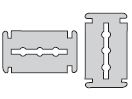##### Question 6:

If $∆$ABC $\cong$ $∆$FED under the correspondence ABC$↔$ FED, write all the corresponding congruent parts of the triangles.

If $∆$ABC $\cong$ $∆$FED under the correspondence ABC$↔$FED it follows that congruent parts (i.e., the matching parts) of congruent triangles are equal.
Therefore all the corresponding congruent parts of the triangles are:
AB = FE, BC = ED and AC = FD $\angle$A = $\angle$F, $\angle$B = $\angle$E and $\angle$C = $\angle$D

##### Question 7: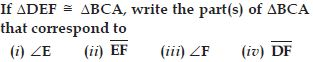$∆$DEF $\cong$ $∆$BCA. Therefore the correspondence is DEF$↔$BCA.
For better understanding of the correspondence let us use a diagram.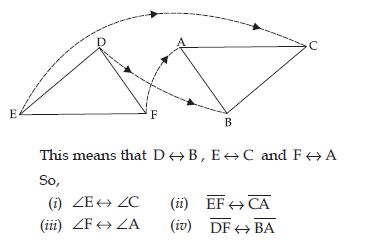##### Question 8:

In fig., lengths of the sides of the triangles are indicated. By applying the SSS congruence rule, state which pairs of triangles are congruent. In case of congruent triangles, write the result in symbolic form: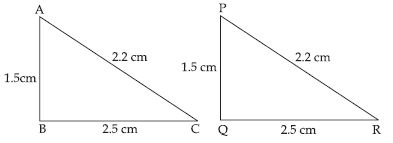In $∆$ABC and $∆$PQR, we have;
AB = PQ (= 1.5), BC = QR (= 2.5) and AC = PR = 2.2
This shows that the three sides of $∆$ABC are equal to the three sides of $∆$PQR.
So, by SSS congruence rule two triangles are congruent.
The correspondence is A$↔$P , B$↔$Q and C$↔$R.
So, in symbolic form $∆$ABC $\cong$ $∆$PQR

##### Question 9:

In fig., lengths of the sides of the triangles are indicated. By applying the SSS congruence rule, state which pairs of triangles are congruent. In case of congruent triangles, write the result in symbolic form: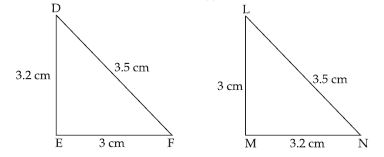In $∆$DEF and $∆$LMN, we have;
DE = MN (= 3.2), EF = LM (= 3) and DF = LN (= 3.5)
This shows that the three sides of $∆$DEF are equal to the three sides of $∆$LMN.
So, by SSS congruence rule two triangles are congruent.
The correspondence is D$↔$N, E$↔$M and F$↔$L.
So, in symbolic form $∆$DEF $\cong$ $∆$NML

##### Question 10:

In fig., lengths of the sides of the triangles are indicated. By applying the SSS congruence rule, state which pairs of triangles are congruent. In case of congruent triangles, write the result in symbolic form: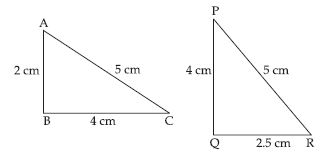In $∆$ABC and $∆$PQR, we have
BC = PR (= 4), AC = PQ (= 5)
But AB $\ne$ QR [ $\because$ 2 $\ne$ 2.5]
So, SSS congruence rule does not applicable.
Therefore $∆$ABC is not congruent to $∆$PQR.

##### Question 11:

In fig., lengths of the sides of the triangles are indicated. By applying the SSS congruence rule, state which pairs of triangles are congruent. In case of congruent triangles, write the result in symbolic form: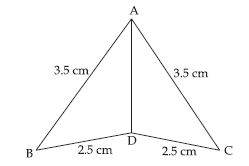In $∆$ABC and $∆$ADC, we have AB = AC (= 3.5), BD = DC (= 2.5) and AD = AD This shows that the three sides of $∆$ABD are equal to the three sides of $∆$ADC.
So, by SSS congruence rule two triangles are congruent.
The correspondence is A$↔$A , B$↔$C and D$↔$D.
So; in symbolic form $∆$ABD $\ne$ $∆$ACD

##### Question 12: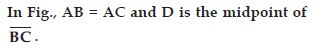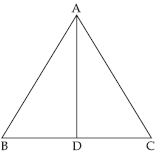State the three pairs of equal parts in $∆$ADB and $∆$ADC.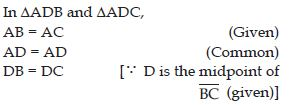##### Question 13:Is $∆$ADB $\cong$ $∆$ADC? Give reasons.

Yes; $∆$ADB $\cong$ $∆$ADC.
Reason: In $∆$ADB and $∆$ADC,
AB = AC (Given)
DB = DC [$\because$ D is the midpoint of BC (given)]
We observe that three sides of $∆$ADB are equal to the three sides of $∆$ADB are equal to the three sides of $∆$ADC.
So, by SSS congruence rule two triangles are congruent.
The congrunece is A$↔$A , D$↔$D and B$↔$C
So, $∆$ADB $\cong$ $∆$ADC

##### Question 14:Is $\angle$B = $\angle$C? Why?

Yes; $\angle$B = $\angle$C
Reason: In $∆$ADB and $∆$ADC,
AB = AC (Given)
DB = DC [$\because$ D is the midpoint of BC (given)]
We observe that three sides of $∆$ADB are equal to the three sides of $∆$ADB are equal to the three sides of $∆$ADC.
So, by SSS congruence rule two triangles are congruent.
The congrunece is A$↔$A , D$↔$D and B$↔$C
So, $∆$ADB $\cong$ $∆$ADC
Thus B corresponds to C (i.e. B$↔$C ) corresponding parts of congruent triangles are equal.
$\therefore$ $\angle$B = $\angle$C

##### Question 15:

In fig., AC = BC and AD = BC. Which of the following statements is meaningfully written?
(i) $∆$ABC $\cong$ $∆$ABD
(ii) $∆$ABC $\cong$ $∆$BAD.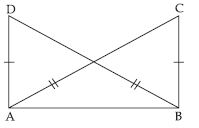In $∆$ABC and $∆$ABD,
AC = BD (given)
and AB = AB (common)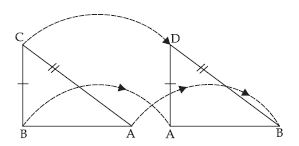This shows that the three sides of $∆$ABC are equal to the three sides of $∆$ABD.
So, by SSS congruence rule two triangles are congruent.
The correspondence is A$↔$B , B$↔$A and C$↔$D .
So in symbolic form, $∆$ABC $\cong$ $∆$BAD
Hence, statement (ii) i.e., $∆$ABC $\cong$ $∆$BAD is meaningfully written.

##### Question 16:

Draw two isosceles triangle ABC with AB = AC.
State the three pairs of equal parts in $∆$ABC and $∆$ACB.

Three pairs of equal parts in $∆$ABC and $∆$ACB are as follows:
In $∆$ABC and $∆$ACB,
AB = AC (given)
BC = CB (same side of both triangles)
AC = AB (given)

##### Question 17:

Draw two isosceles triangle ABC with AB = AC.
Is $∆$ABC $\cong$ $∆$ACB? Why or why not?

Yes; $∆$ABC $\cong$ $∆$ACB by SSS congruence
In $∆$ABC and $∆$ACB,
AB = AC (given)
BC = CB (same side of both triangles)
AC = AB (given)
Therefore, $∆$ABC $\cong$ $∆$ACB by SSS congruence

##### Question 18:

Draw two isosceles triangle ABC with AB = AC.
Is $\angle$B = $\angle$C? Why or why not?

Yes; $\angle$B = $\angle$C
In $∆$ABC and $∆$ACB,
AB = AC (given)
BC = CB (same side of both triangles)
AC = AB (given)
Therefore, $∆$ABC $\cong$ $∆$ACB by SSS congruence
$\therefore$ $\angle$B = $\angle$C
[Congruent parts of congruent triangles.]

##### Question 19: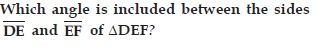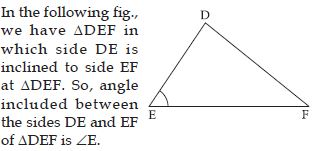##### Question 20:

By applying SAS congruence rule, you want to establish that $∆$PQR $\cong$ $∆$FED. It is given that PQ = FE and RP = DF. What additional information is needed to establish the congruence?

In $∆$PQR and $∆$FED; we are given equality of two
corresponding sides as PQ = FE and RP = DF.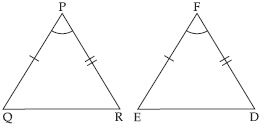To establish $∆$PQR $\cong$ $∆$FED under SAS
congruence we need the equality.
Angles included between the sides PQ and RR,
FE and DF.
So, included $\angle$P should be equal to included $\angle$F.

##### Question 21:

In fig., measures of some parts of the triangles are indicated. By applying SAS congruence rule, state the pairs of congruent triangles, if any, in each case. In case of congruent triangles, write them in symbolic form.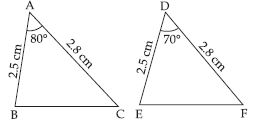In $∆$ABC and $∆$DEF, we have
AB = DE (= 2.5 cm) and AC = DF (= 2.8 cm)
but included $\angle$A $\ne$ included $\angle$D
[ $\angle$A = 80$°$ and $\angle$D = 70$°$ and 80$°$ $\ne$ 70$°$]
So, SAS congruence rule does not applicable.
Therefore $∆$ABC is not congruent to $∆$DEF.

##### Question 22:

In fig., measures of some parts of the triangles are indicated. By applying SAS congruence rule, state the pairs of congruent triangles, if any, in each case. In case of congruent triangles, write them in symbolic form.In $∆$ABC and $∆$RPQ, we have ;
AC = RP (= 2.5 cm), BC = PQ (= 3 cm) and included $\angle$C = included $\angle$P (= 35$°$) So, by SAS congruence rule two triangles are congruent.
The correspondence is
A$↔$R , C$↔$$\angle$P and $\angle$B $↔$Q
So, in symbolic form $∆$ABC $\cong$ $∆$RQP.

##### Question 23:

In fig., measures of some parts of the triangles are indicated. By applying SAS congruence rule, state the pairs of congruent triangles, if any, in each case. In case of congruent triangles, write them in symbolic form.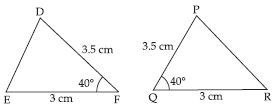$∆$DEF and $∆$PQR
EF = QR (= 3 cm) and DF = PR (= 3.5 cm)
But included $\angle$F $\ne$ included $\angle$R
[$\because$ $\angle$F = 40$°$ given but measure of $\angle$R is not given as 40$°$]
So, SAS congruence rule does not applicable.
Therefore $∆$DEF is not congruent to $∆$PQR.

##### Question 24:

In fig., measures of some parts of the triangles are indicated. By applying SAS congruence rule, state the pairs of congruent triangles, if any, in each case. In case of congruent triangles, write them in symbolic form.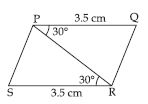In fig. PQRS is a parallelogram. Diagonal PR divides it into two triangles $∆$PQR and $∆$PRS.
Now, in $∆$PQR and $∆$PRS, we have PQ = SR (= 3.5 cm) PR = PR (common) and included $\angle$P = included $\angle$R (= 30$°$).
So, by SAS congruence rule two triangles are congruent.
The correspondence is P$↔$R , Q$↔$ S , R$↔$P So, in symbolic form $∆$PQR $\cong$ $∆$RSP.

##### Question 25: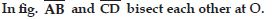State the three pairs of equal parts in two triangles AOC and BOD.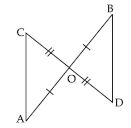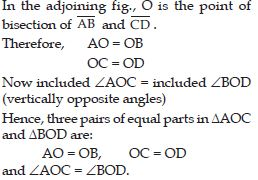##### Question 26:Which of the following statements are true?
(a) $∆$AOC $\cong$ $∆$DOB
(b) $∆$AOC$\cong$ $∆$BOD

In $∆$AOC and $∆$BOD
AO = OB,
OC = OD and
included $\angle$AOC = included $\angle$BOD.
This shows that by SAS congruence rule two triangles are congruent.
The correspondence is A$↔$B , O$↔$O , C$↔$D
So in symbolic form $∆$AOC $\cong$ $∆$BOD
Hence, statement (b) i.e. $∆$AOC $\cong$ $∆$BOD is true.

##### Question 27:

What is the side included between the angles
M and N of $∆$MNP?

The side included between the angles M and N of $∆$MNP are MN.

##### Question 28:

You want to establish $∆$DEF $\cong$ $∆$MNP, using the ASA congruence rule. You are given that $\angle$D = $\angle$M and $\angle$F = $\angle$P. What information is needed to establish the congruence? (Draw a rough figure and then try !)

We want to establish $∆$DEF $\cong$ $∆$MNP, using the ASA congruence rule for this, we have given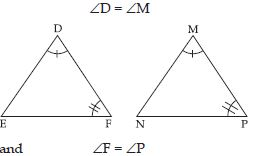Also, required included sides are DF = MP

##### Question 29:

In fig., measures of some parts are indicated.
By applying ASA congruence rule, state which pairs of triangles are congruent. In case of congruence, write the result in symbolic form.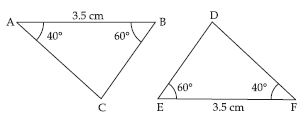Yes, $∆$ABC $\cong$ $∆$DEF
Reason: In $∆$ABC and $∆$FED,
$\angle$A = $\angle$F = 40$°$
AB = EF = 3.5 cm
$\angle$B = $\angle$E = 60$°$
$\therefore$ By ASA congruence rule,
$∆$ABC $\cong$ $∆$FED

##### Question 30:

In fig., measures of some parts are indicated.
By applying ASA congruence rule, state which pairs of triangles are congruent. In case of congruence, write the result in symbolic form.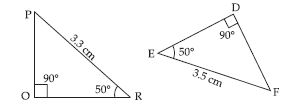No, given triangles are not congruent.

##### Question 31:

In fig., measures of some parts are indicated.
By applying ASA congruence rule, state which pairs of triangles are congruent. In case of congruence, write the result in symbolic form.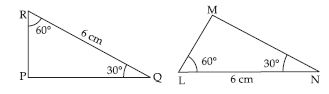Yes, $∆$PRQ $\cong$ $∆$MLN
Reason: In $∆$RPQ and $∆$LMN,
$\angle$R = $\angle$L = 90$°$
RQ = LN = 6 cm
$\angle$Q = $\angle$N = 30$°$
$\therefore$ By ASA congruence rule,
$∆$RPQ $\cong$ $∆$LMN

##### Question 32:

In fig., measures of some parts are indicated.
By applying ASA congruence rule, state which pairs of triangles are congruent. In case of congruence, write the result in symbolic form.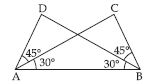Yes, $∆$DAB $\cong$ $∆$CAB,
Reason: In $∆$DAB and $∆$CBA
$\angle$DAB = $\angle$CBA = 45$°$ + 30$°$ = 75$°$
AB = BA (Common side)
$\angle$DBA = $\angle$CAB = 30$°$
$\therefore$ By ASA congruence rule,
$∆$DAB $\cong$ $∆$CAB

##### Question 33:

Given below are measurements of some parts of two triangles. Examine whether the two triangles are congruent or not, by ASA congruence rule. In case of congruence, write it in symbolic form.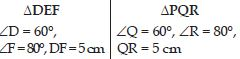Yes, $∆$DEF $\cong$ $∆$PQR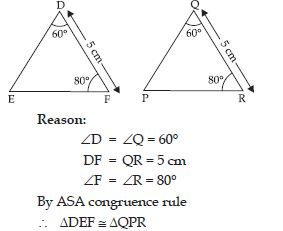##### Question 34:

Given below are measurements of some parts of two triangles. Examine whether the two triangles are congruent or not, by ASA congruence rule. In case of congruence, write it in symbolic form.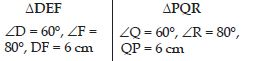No, $∆$DEF is not congruent to $∆$PQR.

##### Question 35:

Given below are measurements of some parts of two triangles. Examine whether the two triangles are congruent or not, by ASA congruence rule. In case of congruence, write it in symbolic form.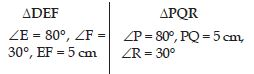No, $∆$DEF is not congruent to $∆$PQR.

##### Question 36:

In fig., ray AZ bisects $\angle$DAB as well as $\angle$DCB.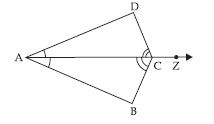State the three pairs of equal parts in triangles BAC and DAC.

Three pairs of equal parts in triangles BAC and DAC are:
$\angle$BAC = $\angle$DAC [$\because$ AZ bisects $\angle$DAB]
$\angle$BCA = $\angle$DCA [$\because$ AZ bisects $\angle$DCB]
AC = CA (Common side)

##### Question 37:

In fig., ray AZ bisects $\angle$DAB as well as $\angle$DCB.Is $∆$BAC $\cong$ $∆$DAC? Give reasons.

In $∆$BAC and $∆$DAC
$\angle$BAC = $\angle$DAC [$\because$ AZ bisects $\angle$DAB]
$\angle$BCA = $\angle$DCA [$\because$ AZ bisects $\angle$DCB]
AC = CA (Common side)
By ASA congruence rule,
$∆$BAC $\cong$ $∆$DAC

##### Question 38:

In fig., ray AZ bisects $\angle$DAB as well as $\angle$DCB.Reason:
In $∆$BAC and $∆$DAC
$\angle$BAC = $\angle$DAC [$\because$ AZ bisects $\angle$DAB]
$\angle$BCA = $\angle$DCA [$\because$ AZ bisects $\angle$DCB]
AC = CA (Common side)
By ASA congruence rule,
$∆$BAC $\cong$ $∆$DAC
$\therefore$ AB = AD (CPCT)

##### Question 39:

In fig., ray AZ bisects $\angle$DAB as well as $\angle$DCB.Is CD = CB? Give reasons.

Yes, CD = CB
Reason:
In $∆$BAC and $∆$DAC
$\angle$BAC = $\angle$DAC [$\because$ AZ bisects $\angle$DAB]
$\angle$BCA = $\angle$DCA [$\because$ AZ bisects $\angle$DCB]
AC = CA (Common side)
By ASA congruence rule,
$∆$BAC $\cong$ $∆$DAC
$\therefore$ CD = CB (CPCT)

##### Question 40:

In fig, measures of some parts of triangles are given. By applying RHS congruence rule, state which pairs of triangles are congruent. In case of congruent triangles, write the result in symbolic form.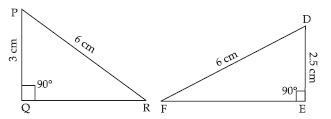No, $∆$PQR is not congruent to $∆$DEF.
Yes, $∆$CAB $\cong$ $∆$DAB
Reason:
$\angle$C = $\angle$D = 90$°$
AB = AB = 3.5 cm
CA = DB = 2 cm
$\therefore$ By RHS congruence rule,
$∆$CAB $\cong$ $∆$DAB

##### Question 41:

In fig, measures of some parts of triangles are given. By applying RHS congruence rule, state which pairs of triangles are congruent. In case of congruent triangles, write the result in symbolic form.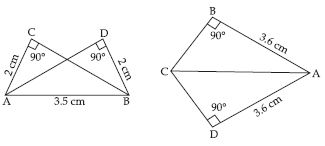Yes, $∆$ABC $\cong$ $∆$ADC
Reason:
$\angle$B = $\angle$D = 90$°$
CA = CA (Common side)
BA = DA = 3.6 cm
$\therefore$ By RHS congruence rule,
$∆$ABC $\cong$ $∆$ADC

##### Question 42:

In fig, measures of some parts of triangles are given. By applying RHS congruence rule, state which pairs of triangles are congruent. In case of congruent triangles, write the result in symbolic form.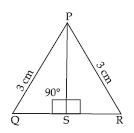Yes, $∆$PSQ $\cong$ $∆$PSR
Reason:
$\angle$PSQ = $\angle$PSR = 90$°$
PQ = PR = 3 cm
PS = PS (Common side)
$\therefore$ By RHS congruence rule,
$∆$PSQ $\cong$ $∆$PSR

##### Question 43:

It is to be established by RHS congruence rule that $∆$ABC $\cong$ $∆$RPQ. What additional information is needed, if it is given that: $\angle$B = $\angle$P = 90$°$ and AB = RP?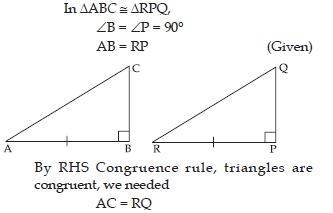##### Question 44:

In fig., BD and CE are altitudes of $∆$ABC such that BD = CE.
State the three pairs of equal parts in $∆$CBD and $∆$BCE.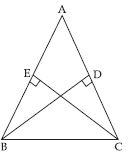Three pairs of equal parts in $∆$CBD and $∆$BCE are:
$\angle$D = $\angle$E = 90$°$
CB = BC (Common side)
BD = CE (Given)

##### Question 45:

In fig., BD and CE are altitudes of $∆$ABC such
that BD = CE.
Is $∆$CBD $\cong$ $∆$BCE? Why or why not?Yes, $∆$CBD $\cong$ $∆$BCE.
Reason:
In $∆$CBD and $∆$BCE
$\angle$D = $\angle$E = 90$°$
CB = BC (Common side)
BD = CE (Given)
By RHS Congruence rule,
$\therefore$ $∆$CBD $\cong$ $∆$BCE

##### Question 46:

In fig., BD and CE are altitudes of $∆$ABC such
that BD = CE.
Is $\angle$DCB = $\angle$EBC? Why or why not?Yes, $\angle$DCB $\cong$ $\angle$EBC
Reason:
In $∆$CBD and $∆$BCE
$\angle$D = $\angle$E = 90$°$
CB = BC (Common side)
BD = CE (Given)
By RHS Congruence rule,
$\therefore$ $∆$CBD $\cong$ $∆$BCE
$\therefore$ $\angle$DCB = $\angle$EBC (CPCT)

##### Question 47:

ABC is an isosceles triangle with AB = AC and AD is one of its altitudes (fig.).
State the three pairs of equal parts in $∆$ADB and $∆$ADC.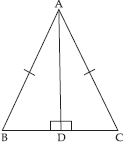Three pairs of equal parts in $∆$ADB and $∆$ADC are:
$\angle$ADB = $\angle$ADC = 90$°$
AB = AC (Given)

##### Question 48:

ABC is an isosceles triangle with AB = AC and AD is one of its altitudes (fig.).
Is $∆$ADB $\cong$ $∆$ADC? Why or why not?Yes, $∆$ADB $\cong$ $∆$ADC
Reason:
In $∆$ADB and $∆$ADC
$\angle$ADB = $\angle$ADC = 90$°$
AB = AC (Given)
By RHS Congruence rule,
$\therefore$ $∆$ADB $\cong$ $∆$ADC

##### Question 49:

ABC is an isosceles triangle with AB = AC and AD is one of its altitudes (fig.).
Is $\angle$B = $\angle$C? Why or why not?Yes, $\angle$B = $\angle$C
Reason:
In $∆$ADB and $∆$ADC
$\angle$ADB = $\angle$ADC = 90$°$
AB = AC (Given)
By RHS Congruence rule,
$\therefore$ $∆$ADB $\cong$ $∆$ADC
$\therefore$ $\angle$B = $\angle$C (CPCT)

##### Question 50:

ABC is an isosceles triangle with AB = AC and AD is one of its altitudes (fig.).
Is BD = CD? Why or why not?Yes, BD $\cong$ CD
Reason:
In $∆$ADB and $∆$ADC
$\angle$ADB = $\angle$ADC = 90$°$
AB = AC (Given)
By RHS Congruence rule,
$\therefore$ $∆$ADB $\cong$ $∆$ADC
$\therefore$ BD = CD (CPCT)

##### Question 51:

Which congruence criterion do you use in the following?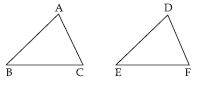Given: AC = DF
AB = DE
BC = EF
So, $∆$ABC $\cong$ $∆$DEF

In $∆$ABC and $∆$DEF,
Given that,
AC = DF
AB = DE
and BC = EF
So, $∆$ABC $\cong$ $∆$DEF
By SSS congruence criterion.

##### Question 52:

Which congruence criterion do you use in the following?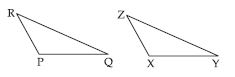Given: ZX = RP
RQ = ZY
$\angle$PRQ = $\angle$XZY
So, $∆$PQR $\cong$ $∆$XYZ

In $∆$RPQ and $∆$ZXY,
Given that,
RP = ZX
RQ = ZY
and $\angle$PRQ = $\angle$XZY
So, $∆$PQR $\cong$ $∆$XYZ
By SAS congruence criterion.

##### Question 53:

Which congruence criterion do you use in the following?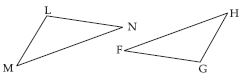Given: $\angle$MLN = $\angle$FGH
$\angle$NML = $\angle$GFH
ML = FG
So, $∆$LMN $\cong$ $∆$GFH

In $∆$LMN and $∆$GFH,
Given that,
$\angle$MLN = $\angle$FGH
$\angle$NML = $\angle$GFH
ML = FG
So, $∆$LMN $\cong$ $∆$GFH
By ASA congruence criterion.

##### Question 54:

Which congruence criterion do you use in the following?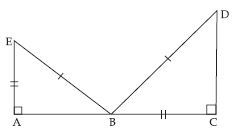Given: EB = DB
AE = BC
$\angle$A = $\angle$C = 90$°$
So, $∆$ABE $\cong$ $∆$CDB

In $∆$ABE and $∆$CDB,
Given that,
EB = DB
AE = BC
$\angle$A = $\angle$C = 90$°$
So, $∆$ABE $\cong$ $∆$CDB
By RHS congruence criterion.

##### Question 55:

You want to show that $∆$ART $\cong$ $∆$PEN,
If you have to use SSS criterion, then you need to show
(i) AR = (ii) RT = (iii) AT =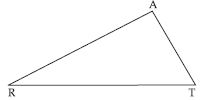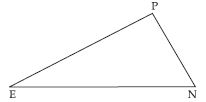$∆$ART $\cong$ $∆$PEN (Given)
By SSS criterion, then
(i) AR = PE
(ii) RT = EN
(iii) AT = PN

##### Question 56:

You want to show that $∆$ART $\cong$ $∆$PEN,
If it is given that $\angle$T = $\angle$N and you are to use SAS criterion, you need to have
(i) RT = and (ii) PN =$∆$ART $\cong$ $∆$PEN [Given]
and $\angle$T = $\angle$N
Using SAS criterion, then
(i) RT = EN
(ii) PN = AT

##### Question 57:

You want to show that $∆$ART $\cong$ $∆$PEN,
If it is given that AT = PN and you are to use ASA criterion, you need to have:
(i) ? (ii) ?$∆$ART $\cong$ $∆$PEN (Given)
By using ASA criterion,
Also, AT = PN (Given)
(i) $\angle$RAT = $\angle$EPN
(ii) $\angle$ATR = $\angle$PNE

##### Question 58:

You have to show that $∆$AMP $\cong$ $∆$AMQ. In the
following proof, supply the missing reasons.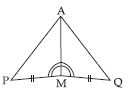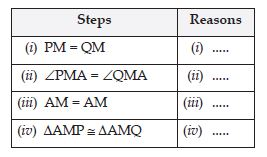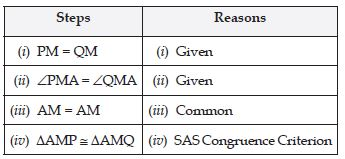##### Question 59:

In $∆$ABC, $\angle$A = 30$°$, $\angle$B = 40$°$ and $\angle$C = 110$°$
In $∆$PQR, $\angle$P = 30$°$, $\angle$Q = 40$°$ and $\angle$R = 110$°$
A student says that $∆$ABC $\cong$ $∆$PQR by AAA congruence criterion. Is he justified? Why or why not?

No, AAA congruence criterion is not sufficient to say that two triangles are congruent because angle shows the direction (or inclination) of any triangle and not its size.

##### Question 60:

In the figure, the two triangles are congruent. The corresponding parts are marked. We can write $∆$RAT $\cong$ ?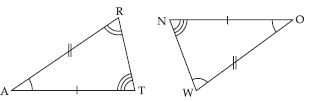Given that, AT = ON
AR = OW
$\angle$A = $\angle$O
$\angle$R = $\angle$W
and $\angle$T = $\angle$N
then $∆$RAT $\cong$ $∆$WON

##### Question 61:

Complete the congruence statement: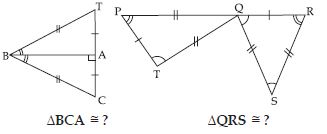(i) Given that BT = BC
AT = AC
$\angle$TBA = $\angle$CBA
$\therefore$ $∆$BCA $\cong$ $∆$BTA
(ii) Given that,
$\angle$P = $\angle$R
$\angle$T = $\angle$S
PT = QR
$\therefore$ $∆$QRS $\cong$ $∆$TPQ

##### Question 62:

In a squared sheet, draw two triangles of equal areas such that
(i) the triangles are congruent.
(ii) the triangles are not congruent.
What can you say about their perimeters?

In a square sheet, draw two triangles of equal areas such that:
(i) the triangles are congruent, then their perimeters are equal.
(ii) the triangles are not congruent, then their perimeters are not equal.

##### Question 63:

Draw a rough sketech of two triangles such that they have five pairs of congruent parts but still the triangles are not congruent.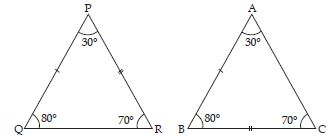Here, $\angle$P = $\angle$A, $\angle$Q = $\angle$B, $\angle$R = $\angle$C
Also, PQ = AB, PR = BC

##### Question 64:

If $∆$ABC and $∆$PQR are to be congruent, name one additional pair of corresponding parts. What criterion did you use?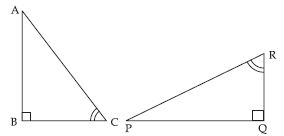For $∆$ABC and $∆$PQR to be congruent we must need BC = QR
$\because$ $\angle$B = $\angle$Q = 90$°$
and $\angle$C = $\angle$R (Given)
By ASA congruence criterion,
$\therefore$ $∆$ABC $\cong$ $∆$PQR

##### Question 65:

Explain, why $∆$ABC $\cong$ $∆$FED.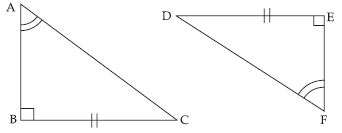$\angle$A = $\angle$F
and $\angle$B = $\angle$E
$\angle$A + $\angle$B = $\angle$F + $\angle$E
or 180$°$ – ($\angle$A + $\angle$B) = 180$°$ – ($\angle$F + $\angle$E)
or $\angle$C = $\angle$D
Now, $\angle$B = $\angle$E = 90$°$
and $\angle$C = $\angle$D (Proved)
$∆$ABC ≅ $∆$FED